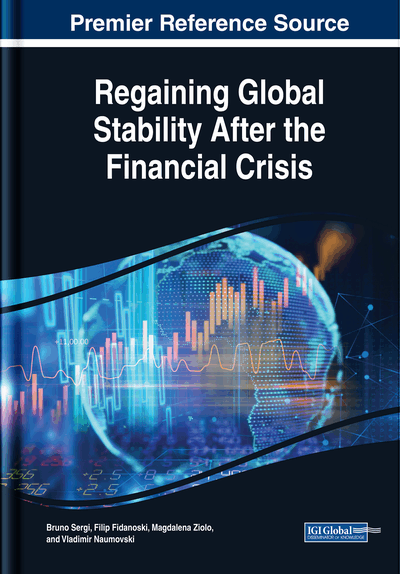# Dynamic Risk Management of Investment Portfolio by Futures Contracts

Alexander K. Kerimov, Oleg I. Pavlov
DOI: 10.4018/978-1-5225-4026-7.ch008
OnDemand:
(Individual Chapters)
Available
\$29.50
No Current Special Offers

## Abstract

This chapter is devoted to the dynamic risk management of the investment portfolio using future contracts. The number of futures for each portfolio asset, which is determined by portfolio effectiveness and acceptability of risk at each step, serves as a control parameter. The authors define effective portfolios as the ones of the minimum variance with the expected return greater than or equal to the specified value. Risk is measured by the probability of losing a certain part of the portfolio value. Effective adaptive strategies of portfolio risk management are proposed and their comparative analysis is carried out on a concrete example. In order to determine risk management strategies, the authors implement simple methods of volatility forecasting and correlation of relative changes of price data based on exponential moving average.
Chapter Preview
Top

## Introduction And Background

Risk management method of the investment portfolio that utilizes future contracts is based on a strong positive correlation between changes in the spot price and the future price for a given asset. The main issue of insurance is the estimation of the necessary number of futures contracts needed for insurance and the assessment of the effectiveness of insurance over a given period (Brown (2001); Walsh (1995); Ederington (1979); Haustalter (2000); Hull (2017); Bhargava and Brooks (2001); Petersen and Thiagarajan (2000)). The traditional method of estimating the optimal number of futures contracts involves estimation of regression of the spot price change on changes in the futures price. The coefficient at the futures price changes in this regression determines the necessary number of futures contracts that minimize the variance of inhomogeneous portfolios. In case of stock portfolio, one can use regression of the portfolio value on a market index.

This method has the following shortcomings. Time series of changes in the spot price and the future price are normally inhomogeneous with respect to the mean and variance. Instability of the mean leads to an inadequate forecast of the expected return. Instability of the variance (heteroscedasticity) leads to significant distortion of the hedge coefficient. Besides, the correlation coefficient between changes in the spot price and the future price varies in time. Usually, it increases significantly as expiration date approaches. Moreover, due to nonstationarity of financial time series, the traditional correlation estimation that is based on a simple averaging is often incorrect or results in problems with sample size calculation. All this makes it harder to obtain a correct estimation of the number of futures contracts that hedge a position for an asset using traditional econometric methods (see Dark (2005)).

Furthermore, the traditional approach for determining the required number of futures contracts takes into account only one parameter - variance, which characterizes price variability of a portfolio. So, such important portfolio parameters as the expected return or possible restrictions on the number of futures contracts are not taken into account. Thus, the possible additional profit through the use of derivative instruments is not taken into account

We employ stochastic control for dynamic risk-management of positions by futures contracts on the basis of effective inhomogeneous portfolios (see Kerimov (2014), Cecchetti et al. (1998)). The effective portfolios are defined as the ones of the minimum variance with the expected return greater than or equal to the specified value. In turn, we select this value in such a way that the probability of a certain level of loss is minimal or does not exceed a predefined value at each step.

The control parameters used are the number of futures for each underlying asset of portfolio. Proposed approach provides the correction of forecasts of probabilistic characteristics of an inhomogeneous portfolio as the new data is received. An essential aspect of this approach is the interpretation of financial time series as a random process, rather than a random variable. With this approach, forecasts and standard deviations of forecasts are corrected, as the new data become available (Engle (1982), Cumby et al. (1993); Kroner and Sultan (1993)). Usually, analysis and forecast of time series is a rather complicated procedure. However, the effectiveness of the dynamic risk-management is largely determined by the adequacy of forecasts and their confidence intervals. We use a fairly simple but quite satisfactory method of adaptive forecasting based on exponential moving average.

## Complete Chapter List

Search this Book:
Reset